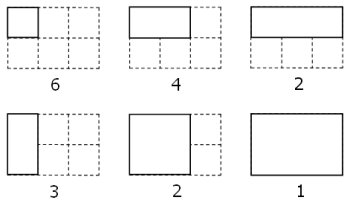2015-10-30

# Problem 086: Cuboid route

Description:

A spider, S, sits in one corner of a cuboid room, measuring `6 by 5 by 3`, and a fly, F, sits in the opposite corner. By travelling on the surfaces of the room the shortest "straight line" distance from S to F is 10 and the path is shown on the diagram.However, there are up to three "shortest" path candidates for any given cuboid and the shortest route doesn't always have integer length.

It can be shown that there are exactly `2060` distinct cuboids, ignoring rotations, with integer dimensions, up to a maximum size of `M by M by M`, for which the shortest route has integer length when `M = 100`. This is the least value of M for which the number of solutions first exceeds two thousand; the number of solutions when `M = 99` is `1975`.

Find the least value of M such that the number of solutions first exceeds one million.

Solution:
v##### ##                                    >       >\$30gv >`#v_v
>"}}@"**50p040p0>1+:v>>1-::*70g:*+v  vp01g03_^#-g01:_^#- <: g  : :
:+  v         <  >:30p:20 g\/+2/: 30g^* 0>#1v2
71 >           >#^ #p #0 2 #<\$      v   7gv+</
0*  >:88*%"9"`#^_:88+%:9`#v_:2-#v_v   2 :082g8
p2      >     >     >     > #     >\$>\$0>|80/0
0p |!-8_^#-7:_^#-6:_^#-5:_^# -3:<     g  +p7^<
80            >     ^<   0 <^:p010 <^<-! ^10#<
>            ^   >^ >:55+%:7-!#^_:3-!#^_2-!#^_:3%2-#^_^>^  \$g -
@._^#`g05p04:+g04g08\$_^#!:                                  < <>\^
Start
??
Pause
Reset
Output:
Stack:   (0)

Explanation:

The spider essentially travels on the hypotenuse of a triangle with the sides `A` and `B+C`. For it to be the shortest path the condition `A <= B+C` must be true.

The amount of integer-cuboids for a given value M is "All integer-cuboids with `A=M`" plus integer-cuboids(M-1). In our loop we start with `M=1` and increment it in every step. We also remember the last value (for `M-1`) and loop until the value exceeds one million.

For a given value `A = M` we go through all possible `BC` value (`0 <= BC <= 2*A`) and test if `M^2 + BC^2` is an integer-square. If such a number is found and `BC <= A` then this means we have found `BC/2` additional cuboids (there are `BC/2` different `B+C = BC` combinations where `B <= C`) If, on the other hand `BC > A` then we have only found `A - (BC + 1)/2 + 1` additional cuboids (because the condition`A <= BC` must be satisfied).

One of the more interesting parts was the `isSquareNumber()` function, which test if a number `x` has an integer square-root. To speed this function up (it takes most of the runtime) we can eliminate around 12% of the numbers with a few clever tricks. For example if the last digit of `x` is `2`, x is never a perfect square-number. Or equally if the last hex-digit is `7`. In our program we test twelve conditions like that:

``````x % 16  > 9
x % 64  > 57
x % 16 == 2
x % 16 == 3
x % 16 == 5
x % 16 == 6
x % 16 == 7
x % 16 == 8
x % 10 == 2
x % 10 == 3
x % 10 == 7
x % 3  == 2``````

Sources:

If none of this pre-conditions is true we have to manually test the number. We use the same the same integer-squareroot algorithm as in previous problems. If `isqrt(x)^2 == x` the we can be sure that x is a perfect square number.

 Interpreter steps: 599 659 030 Execution time (BefunExec): 1min 31s (6.53 MHz) Program size: 66 x 10 (fully conform befunge-93) Solution: 1818 Solved at: 2015-10-30

made with vanilla PHP and MySQL, no frameworks, no bootstrap, no unnecessary* javascript